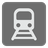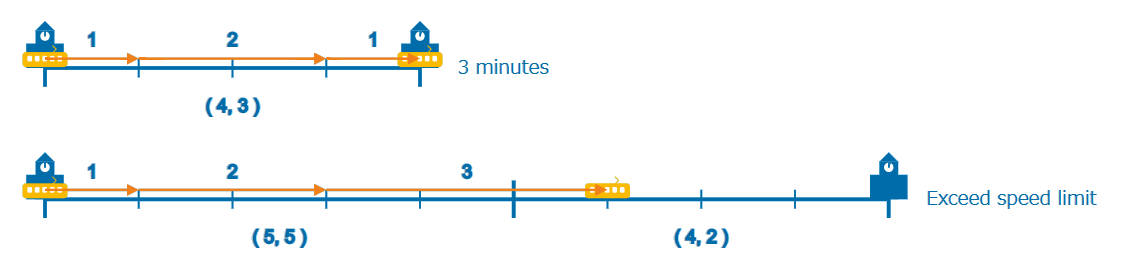Fast Train
Elementary+
English

You are planning the train schedule and you want to know the minimum time of traveling between the stations.

Each section of the rail between stations is given in the Array. Each section is a tuple of distance and speed limit (both are integers). You can change the speed ( +1. -1 and ± 0 ) at the start and every minute after that. The train runs by the same amount as the speed value in a minute.
Note: This means that a train with a speed 2 will travel a distance 2 before another minute passes and its speed can be changed again.

• Starting speed is 0.
• Speed limit is set for each section of the rail. You don't exceed it.
• You must reach the target station at speed 1 (because it’s necessary to stop at the station).

You should return the minimum time (minutes) as an integer.Example:

```fastTrain([[4, 3]]) == 3 // (1, 2, 1)
fastTrain([[9, 5]]) == 5 // (1, 2, 3, 2, 1)
fastTrain([[5, 5], [4, 2]]) == 6 // e.g. (1, 2, 2, 2, 1, 1)
```

Input: An array of the section of the rail. Each section is an array of distance (as an integer) and speed limit (as an integer).

Output: The minimum time (minutes) as an integer.

How it is used:
For efficient acceleration and deceleration.

Precondition:

• distance > 0
• speed limit > 0
• len(section) > 0